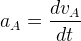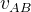## The Postulates of Special Relativity (2)

Suppose in reference frame C, an experiment about Newton’s second law of motion is undergoing. Here we have to prove, given any two reference frames A and B, the observations will lead us to the exact same formula: F=ma. In another word, we must get the same acceleration, because we are observing the same experiment(Both F and m are unique).

In reference frame A, the acceleration is:In reference frame B, the acceleration is:Whereis the relative velocity of reference frame A to reference frame B, which does not change with time, thus leading to.

We can see both reference frames give the same acceleration. Or in another word, Newton’s second law of motion are exactly the same in both reference frames.

Because the reference frames A and B are arbitrarily chosen, one of them might be taken as the reference frame C itself. Thus we can get this further conclusion: the reference frame, where the experiment event is happening, has no effect on the experiment itself, whether it is “at rest” or in uniform motion.

In this way, the validity of the principle of relativity, in the field of mechanical motion, is established.

The above mathematical derivation does not require that both reference frames are in uniform motion, only that they have a constant relative velocity. The requirement for reference frame of uniform motion comes from the validity of Newton’s second law of motion. As the Earth is orbiting the sun and has a self spin, all our experiments are actually performed in reference frames of non-uniform motion.